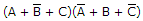# Digital Electronics - Boolean Algebra and Logic Simplification

### Exercise :: Boolean Algebra and Logic Simplification - General Questions

41.

An OR gate with schematic "bubbles" on its inputs performs the same functions as a(n)________ gate.

 A. NOR B. OR C. NOT D. NAND

Answer: Option D

Explanation:

No answer description available for this question. Let us discuss.

42.

Which of the examples below expresses the commutative law of multiplication?

 A. A + B = B + A B. AB = B + A C. AB = BA D. AB = A × B

Answer: Option C

Explanation:

No answer description available for this question. Let us discuss.

43.

Determine the binary values of the variables for which the following standard POS expression is equal to 0.A. (0 + 1 + 0)(1 + 0 + 1) B. (1 + 1 + 1)(0 + 0 + 0) C. (0 + 0 + 0)(1 + 0 + 1) D. (1 + 1 + 0)(1 + 0 + 0)

Answer: Option A

Explanation:

No answer description available for this question. Let us discuss.

44.

The expression W(X + YZ) can be converted to SOP form by applying which law?

 A. associative law B. commutative law C. distributive law D. none of the above

Answer: Option C

Explanation:

No answer description available for this question. Let us discuss.

45.

The commutative law of addition and multiplication indicates that:

 A. we can group variables in an AND or in an OR any way we want B. an expression can be expanded by multiplying term by term just the same as in ordinary algebra C. the way we OR or AND two variables is unimportant because the result is the same D. the factoring of Boolean expressions requires the multiplication of product terms that contain like variables

Answer: Option C

Explanation:

No answer description available for this question. Let us discuss.

#### Current Affairs 2021

Interview Questions and Answers